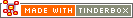^value( expression )^Code Type: Data Include Code Scope of Action: n/a

^value(expression)^

The argument to ^value^ is an expression — a value that could be assigned to an attribute. ^value^ evaluates the expression and exports the result, as a string. This allows manipulations for export use without needing to, for instance, hold the value in a user attribute first.

^value^ is in effect a version of ^get^/^getFor^ but which allows the called attribute's value to be manipulated. For example:

^value(\$Width)^

exports the width of the current note, and is equivalent to

^get(Width)^

So you may use the two forms interchangeably even where no manipulation of the target attribute is required.

More examples:

`^value(sqrt(\$Width))^`

`^value("Name: "+\$Name)^`

`^value(\$Name+":"+\$Name(parent))^`

You don't need to use ^value^ explicitly internally for actions and rules as the expanded v4+ syntax already covers this.

The ^value^ code also gives a method for using action code to retrieve attribute values. The following give the same output:

`^get(SiblingOrder)^` (value here = 10)

`^value(\$SiblingOrder)^` (value here = 10)

 Up: Scope n/a Previous: ^not( condition )^ Next: ^uppercase( data )^

[Last updated: 14 Dec 2009, using v5.0]